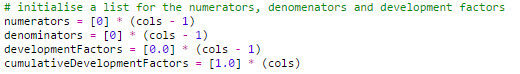top of page
Search

# Chain Ladder Reserving in Python 3

I have been busy the past few weeks but I have found time this evening to post a new article. I’ve noticed that there is a lot of interest in using Python for actuarial work so I thought I would provide a small introduction. In this post I will demonstrate a short Python code which can be used to produce simple actuarial reserve estimates using the basic chain ladder. This is a volume 12 weighted chain ladder method but the code can be adapted to other methods.

This post is a demonstration of what can be done in Python. It is not intended as a guide to reserving or a recommendation of one particular reserving method over another. There are many ways to do this in Python but I have tried to show each step clearly and ensure no step looks like a black box.

The first step is to set up a couple of variables. The cols variables specifies the size of the triangle and the weight variable specifies how many time periods are used for each development factor.Next I define a function which will be used to create an empty list in the shape of a triangle. The first element has a number of sub-elements as set by the size variable. The second element has one less sub-element as so on until the last element which has only one sub element. This is done using two loops, one nested within the other one. The first loop goes down the origin lines of the triangle. As we go down the triangle so the number of times the second loop runs reduces by one on each iteration.

I then call this function to create two empty triangles. One will hold a loss triangle and the other will hold a cumulative loss triangle based on this loss triangle.Then I initialize four more lists. These are going to hold the data required for the development factors. Each element of the numerators list will be divided by each element of the denominators list to create the development factors. Then these will be used to calculate the cumulative development factors. I also declare two more variables, one called ultimate ad one called reserve. These will hold the projected estimate of the ultimate and the reserve.At this step I am going to create an example loss triangle. This is purely for use in this example and is randomly generated. To do this I load in a library called random which has a function called uniform(). This function produces a random number taken from a uniform distribution between two values. The triangle is generated through two loops. The first goes down the origin lines and the second goes across the development columns. If we are in the first development column, then we get a random number between 10,000 and 20,000. If we are in subsequent columns, then we get a random amount which is strictly less than the amount in the column before. Note in the else clause I have used a “\”. This is a special character in Python for continuing the line of code on a new line in the script and is just there to make the code a little tidier.So I fill the empty cumulativeTriangle list with the cumulative values from the loss triangle. Again this is achieved through using loops. The first loop goes down the origin lines, the second loop goes across the development columns. The third loop is a pointer which also goes across the development columns. So the first two loops are used to get to a particular cell in the cumulative triangle and then the pointer goes across the line in the loss triangle accumulating the losses and stops at the relevant column.Now I need to calculate the development factors. To do this I use two lists I created earlier called numerators and denominators. I loop over cells in the cumulative triangle and add the values to the relevant cells in numerators or denominators. The first loop is across the development columns. This goes from the first column and stops at the penultimate column. The second loop runs a pointer down the origin lines in a particular column. It starts at the line corresponding to the penultimate cell in the column minus the weight and adds this to the denominators. It then goes down to the penultimate cell adding the value as it goes. This is repeated in the column immediately to the right but this time starting at the last cell minus the weight and ending at the last cell. These values go into the numerators list. Then with these values stored the development factors are calculated.

There are a lot of ways to do these loops in Python. Including starting at the end and working backwards by using negative indexes.The next step is to obtain the cumulative development factors. Again this is done with a loop across the development periods and a pointer to calculate the product of all the subsequent development periods.Finally, I calculate the ultimate and reserve by applying the development factors and summing the results. This loop goes across the final diagonal of the triangle and multiplies this value by the relevant cumulative development factor.